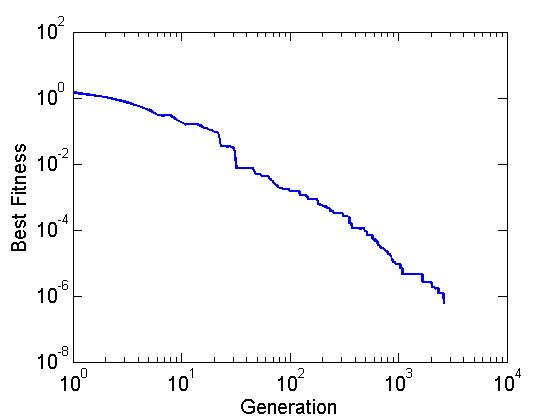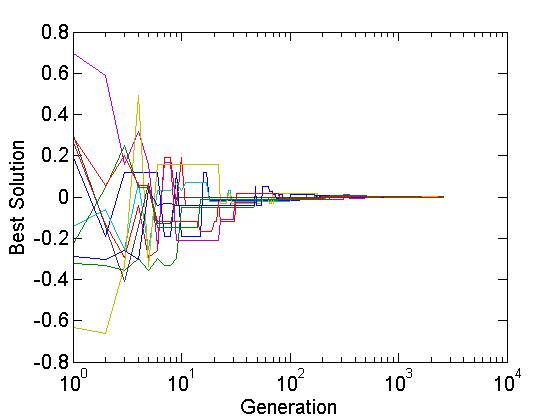13 篇文章 2 订阅

# 遗传算法原理解析

## 与遗传算法有关的生物学概念：

（1）染色体（chromosome）

（2） 个体复制

1. 首先组成一组候选解
2. 依据某些适应性条件测算这些候选解的适应度
3. 根据适应度保留某些候选解，放弃其他候选解
4. 对保留的候选解进行某些操作，生成新的候选解00000|011100000000|10000
11100|000001111110|00101

00000|000001111110|10000
11100|011100000000|00101

000001110000000010000

000001110000100010000

# 遗传算法伪代码

基本遗传算法伪代码
/*
* Pc：交叉发生的概率
* Pm：变异发生的概率
* M：种群规模
* G：终止进化的代数
* Tf：进化产生的任何一个个体的适应度函数超过Tf，则可以终止进化过程
*/

do
{
计算种群Pop中每一个体的适应度F(i)。
初始化空种群newPop
do
{
根据适应度以比例选择算法从种群Pop中选出2个个体
if ( random ( 0 , 1 ) < Pc )
{
对2个个体按交叉概率Pc执行交叉操作
}
if ( random ( 0 , 1 ) < Pm )
{
对2个个体按变异概率Pm执行变异操作
}

} until ( M个子代被创建 )

}until ( 任何染色体得分超过Tf， 或繁殖代数超过G )

## 遗传算法优化方法：

（1）精英主义(Elitist Strategy)选择：是基本遗传算法的一种优化。为了防止进化过程中产生的最优解被交叉和变异所破坏，可以将每一代中的最优解原封不动的复制到下一代中。
（2）插入操作：可在3个基本操作的基础上增加一个插入操作。插入操作将染色体中的某个随机的片段移位到另一个随机的位置。

# 遗传算法实例

function y = my_fitness(population)
% population是随机数[0,1]矩阵，下面的操作改变范围为[-1,1]
population = 2 * (population - 0.5);
y = sum(population.^2, 2); % 行的平方和

function [best_fitness, elite, generation, last_generation] = my_ga( ...
number_of_variables, ...    % 求解问题的参数个数
fitness_function, ...       % 自定义适应度函数名
population_size, ...        % 种群规模（每一代个体数目）
parent_number, ...          % 每一代中保持不变的数目（除了变异）
mutation_rate, ...          % 变异概率
maximal_generation, ...     % 最大演化代数
minimal_cost ...            % 最小目标值（函数值越小，则适应度越高）
)

% 累加概率
% 假设 parent_number = 10
% 分子 parent_number:-1:1 用于生成一个数列
% 分母 sum(parent_number:-1:1) 是一个求和结果（一个数）
% 分子 10     9     8     7     6     5     4     3     2     1
% 分母 10+9+...+1=55
% 相除 0.1818    0.1636    0.1455    0.1273    0.1091    0.0909    0.0727    0.0545    0.0364    0.0182
% 累加(cumsum) 0.1818    0.3455    0.4909    0.6182    0.7273    0.8182    0.8909    0.9455    0.9818    1.0000
% 运算结果可以看出: 累加概率函数是一个从0到1增长得越来越慢的函数
cumulative_probabilities = cumsum((parent_number:-1:1) / sum(parent_number:-1:1)); % 1个长度为parent_number的数列
best_fitness = ones(maximal_generation, 1);% 记录每一代最佳适应度,先初始化为1
elite = zeros(maximal_generation, number_of_variables);% 记录每一代的最优解，初始化为0

% 子女数量=种群数量 - 父母数量（父母即每一代中不发生改变的个体）
child_number = population_size - parent_number; % 每一代子女的数目

% population_size 对应矩阵的行，每一行表示1个个体，行数=个体数（种群数量）
% number_of_variables 对应矩阵的列，列数=参数个数（个体特征由这些参数表示）
population = rand(population_size, number_of_variables);% 初始化种群
last_generation = 0; % 记录跳出循环时的代数

for generation = 1 : maximal_generation % 演化循环开始

% feval把数据带入到一个定义好的函数句柄中计算
% 把population矩阵带入fitness_function函数计算
cost = feval(fitness_function, population); % 计算所有个体的适应度（population_size*1的矩阵）

% index记录排序后每个值原来的行数
[cost, index] = sort(cost); % 将适应度函数值从小到大排序

% index(1:parent_number)
% 前parent_number个cost较小的个体在种群population中的行数
% 选出这部分(parent_number个)个体作为父母，其实parent_number对应交叉概率
population = population(index(1:parent_number), :); % 先保留一部分较优的个体
% population矩阵是不断变化的
% cost在经过前面的sort排序后，矩阵已经改变为升序的
% cost(1)即为本代的最佳适应度
best_fitness(generation) = cost(1); % 记录本代的最佳适应度

% population矩阵第一行为本代的最优解（精英）
elite(generation, :) = population(1, :); % 记录本代的最优解（精英）

% 若本代的最优解已足够好，则停止演化
if best_fitness(generation) < minimal_cost;
last_generation = generation;
break;
end

% 交叉变异产生新的种群，染色体交叉开始
for child = 1:2:child_number % 步长为2是因为每一次交叉会产生2个孩子

% cumulative_probabilities 长度为 parent_number
% 从中随机选择2个父母出来  (child+parent_number)
mother = find(cumulative_probabilities > rand, 1); % 选择一个较优秀的母亲
father = find(cumulative_probabilities > rand, 1); % 选择一个较优秀的父亲

% ceil：向上取整
% rand 生成一个随机数
% 即随机选择了一列，这一列的值交换
crossover_point = ceil(rand*number_of_variables); % 随机地确定一个染色体交叉点

% 假如crossover_point=3, number_of_variables=5
% mask1 = 1     1     1     0     0
% mask2 = 0     0     0     1     1
mask1 = [ones(1, crossover_point), zeros(1, number_of_variables - crossover_point)];

% 获取分开的4段染色体
mother_1 = mask1 .* population(mother, :); % 母亲染色体的前部分
mother_2 = mask2 .* population(mother, :); % 母亲染色体的后部分

father_1 = mask1 .* population(father, :); % 父亲染色体的前部分
father_2 = mask2 .* population(father, :); % 父亲染色体的后部分

% 得到下一代
population(parent_number + child, :) = mother_1 + father_2; % 一个孩子
population(parent_number+child+1, :) = mother_2 + father_1; % 另一个孩子

end % 染色体交叉结束

% 染色体变异开始，变异种群
mutation_population = population(2:population_size, :); % 精英不参与变异，从2开始
number_of_elements = (population_size - 1) * number_of_variables; % 全部基因数目
number_of_mutations = ceil(number_of_elements * mutation_rate); % 变异的基因数目（基因总数*变异率）

% rand(1, number_of_mutations) 生成number_of_mutations个随机数(范围0-1)组成的矩阵(1*number_of_mutations)
% 数乘后，矩阵每个元素表示发生改变的基因的位置（元素在矩阵中的一维坐标）
mutation_points = ceil(number_of_elements * rand(1, number_of_mutations)); % 确定要变异的基因

% 被选中的基因都被一个随机数替代，完成变异
mutation_population(mutation_points) = rand(1, number_of_mutations); % 对选中的基因进行变异操作
population(2:population_size, :) = mutation_population; % 发生变异之后的种群
% 染色体变异结束
end % 演化循环结束

clc,clear all,close all;

% 调用 my_ga 进行计算
% 求解问题的参数个数         10
% 自定义适应度函数名         my_fitness
% 种群规模                  100
% 每一代中保持不变的数目     50 (即交叉率0.5)
% 变异概率                  0.1 (1/10的个体发生变异)
% 最大演化代数              10000 10000代
% 最小目标值                1.0e-6 个体适应度函数值 < 0.000001结束
[best_fitness, elite, generation, last_generation] = my_ga(10, 'my_fitness', 100, 50, 0.1, 10000, 1.0e-6);

% 输出后10行
disp(last_generation);
i_begin = last_generation - 9;
disp(best_fitness(i_begin:last_generation,:));
% disp(best_fitness(9990:10000,:));
% disp(elite(9990:10000,:))
% 不要写成这样，因为GA常常在中间就跳出循环了

% 将elite值转化为问题范围内
my_elite = elite(i_begin:last_generation,:);
my_elite = 2 * (my_elite - 0.5);
disp(my_elite);

% 最佳适应度的演化情况
figure
loglog(1:generation, best_fitness(1:generation), 'linewidth',2)
xlabel('Generation','fontsize',15);
ylabel('Best Fitness','fontsize',15);
set(gca,'fontsize',15,'ticklength',get(gca,'ticklength')*2);

% 最优解的演化情况
figure
semilogx(1 : generation, 2 * elite(1 : generation, :) - 1)
xlabel('Generation','fontsize',15);
ylabel('Best Solution','fontsize',15);
set(gca,'fontsize',15,'ticklength',get(gca,'ticklength')*2);http://blog.jobbole.com/110913/
http://blog.csdn.net/dazhong159/article/details/7908531

http://blog.sciencenet.cn/blog-3102863-1029280.html

https://github.com/Shuai-Xie/genetic-algorithm

https://github.com/yanshengjia/artificial-intelligence/tree/master/genetic-algorithm-for-functional-maximum-problem/src

09-221790
04-2608-24
10-1640万+
03-062万+
09-073万+
05-26427
07-21
07-23978
05-143万+
10-286169
03-301万+
07-267984
12-13438
05-02642
04-25704点击重新获取扫码支付余额充值